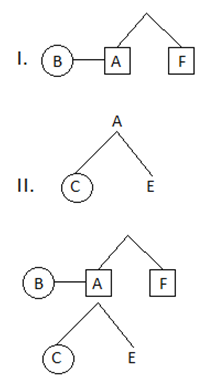# High Level Data Sufficiency Quiz For IBPS PO Part- 7Direction (Q.1-5): Each of the questions below consists of a question and two statements numbered 1 and 2 are given below it. You have to decide whether the data provided in the statements are sufficient to answer the question or not.

### Question.1

Who is the daughter of B?
1. F is the brother of A. A is the husband of B.
2. A has two children C and E. C is the sister of E.

A. The data in Statement 1 alone are sufficient to answer the question, while the data in Statement 2 alone are not sufficient to answer the question.
B. The data in Statement 2 alone are sufficient to answer the question, while the data in Statement 1 alone are not sufficient to answer the question.
C. The data in Statement 1 alone or in Statement 2 alone are sufficient to answer the question.
D. The data in both the Statements 1 and 2 are not sufficient to answer the question.
E. The data in both the Statements 1 and 2 together are necessary to answer the question.
Ans. E.
Solution:### Question..2

There are five persons M, N, O, P and Q in a family. Each of them has a different height. Who is tallest?
1. Q is smaller than only P and N. P is not tallest.
2. P is taller than three. N is taller than Q.

A. The data in Statement 1 alone are sufficient to answer the question, while the data in Statement 2 alone are not sufficient to answer the question.
B. The data in Statement 2 alone are sufficient to answer the question, while the data in Statement 1 alone are not sufficient to answer the question.
C. The data in Statement 1 alone or in Statement 2 alone are sufficient to answer the question.
D. The data in both the Statements 1 and 2 are not sufficient to answer the question.
E. The data in both the Statements 1 and 2 together are necessary to answer the question.
Ans. A.
Solution:
From I = M/O<M/O<Q<P<N

### Question..3

Six persons A, B, C, D, E and F are sitting around a circular table facing towards the centre. Who is the immediate left of F?
1. A is sitting between D and B.
2. B is sitting between A and E. F is sitting immediate left of C.

A. The data in Statement 1 alone are sufficient to answer the question, while the data in Statement 2 alone are not sufficient to answer the question.
B. The data in Statement 2 alone are sufficient to answer the question, while the data in Statement 1 alone are not sufficient to answer the question.
C. The data in Statement 1 alone or in Statement 2 alone are sufficient to answer the question.
D. The data in both the Statements 1 and 2 are not sufficient to answer the question.
E. The data in both the Statements 1 and 2 together are necessary to answer the question.
Ans. D.
Solution:
From I + II = D or E sits immediate left of F.
Because we don't get the exact person who is immediate left of F. So I and II are insufficient to give the answer.

### Question..4

in which of the following date did Shweta not go to office?
1. If Ajay remembered that she did not go to office after 20 but before 25.
2. If Rahul remembered that she took a leave after 23 but before 28.

A. The data in Statement 1 alone are sufficient to answer the question, while the data in Statement 2 alone are not sufficient to answer the question.
B. The data in Statement 2 alone are sufficient to answer the question, while the data in Statement 1 alone are not sufficient to answer the question.
C. The data in Statement 1 alone or in Statement 2 alone are sufficient to answer the question.
D. The data in both the Statements 1 and 2 are not sufficient to answer the question.
E. The data in both the Statements 1 and 2 together are necessary to answer the question.
Ans. E.
Solution:
I. 21, 22, 23, 24
II. 24, 25, 26, 27
From I + II = 24

### Question..5

What is the code for GO?
1. If 'go to office' is written as 'si cha ho' and 'office my house' is written as 'so as si' and 'to went' is written as 'ho ga'.
2. If 'go back home' is written as 'ma na he' and 'home hand above back' is written as 'da na ma ve'.

A. The data in Statement 1 alone are sufficient to answer the question, while the data in Statement 2 alone are not sufficient to answer the question.
B. The data in Statement 2 alone are sufficient to answer the question, while the data in Statement 1 alone are not sufficient to answer the question.
C. The data in Statement 1 alone or in Statement 2 alone are sufficient to answer the question.
D. The data in both the Statements 1 and 2 are not sufficient to answer the question.
E. The data in both the Statements 1 and 2 together are necessary to answer the question.
Ans. C.
Solution:
I. go=cha
II. go =he
Either I or II.